# What is Python and why would I use it?

• Python is an intepreted high-level programming language
• Python is free (as in speech)
• Python runs on most platforms
• It "combines remarkable power with very clear syntax" 
• Well suited for high performance numerical computing (NumPy, ...)
• High quality 2D and 3D visualizations (pylab, mlab, ...)
• Increasingly popular in neuroscience (nipy, nipype, nitime, ...)

# What you should be able to do

## ... in 45mins

• Start Python
• Do simple math
• Get started with linear algebra and scientific computing
• Plot some nice figures

# Use Python for what?

• scripting (like shell scripts, e.g., bash, csh)
• make web sites (like these slides)
• build GUI applications
• science (like Matlab, IDL, R, Octave, Scilab)
• etc.

You just need to know one language to do almost anything !

# Scientific Python building blocks

• Python interpreter: executes Python code
• IPython: an advanced Python shell
• NumPy: provides numerical arrays objects
• SciPy: scientific computing (linear algebra, optimization, regression, etc.)
• Matplotlib a.k.a. Pylab: 2-D visualization, "publication-ready" plots
• Mayavi : 3-D visualization
• Many application specific packages for e.g., machine learning, image processing, symbolic math, ... (an incomplete list)

# First step

Start the IPython shell (from terminal or Windows cmd shell):

```\$ ipython --pylab
```

Getting a scientific-Python environment:

At the Martinos Center:

• See here for how to use scientific-Python on your CentOS workstation

# Hello world!

Start IPython:

```\$ ipython --pylab
```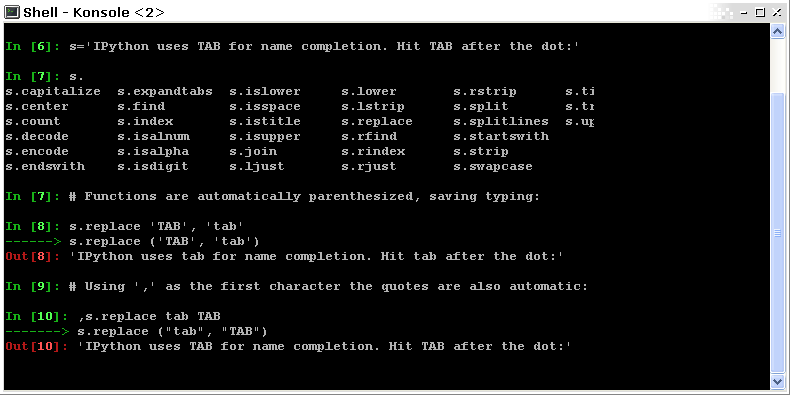Once you have started the interpreter, type:

```>>> print "Hello, world!"
Hello, world!
```

# My first script

Let's say the file my_script.py contains:

```s = 'hello world!'
print s
```

In IPython:

```In : %run my_script.py  # in Matlab just `my_script`
Hello world!

In : s
Out: 'Hello world!'

In : %whos
Variable   Type    Data/Info
----------------------------
s          str     Hello world!
```

# If you are scared of the terminal

..you can use Spyder

```\$ spyder
```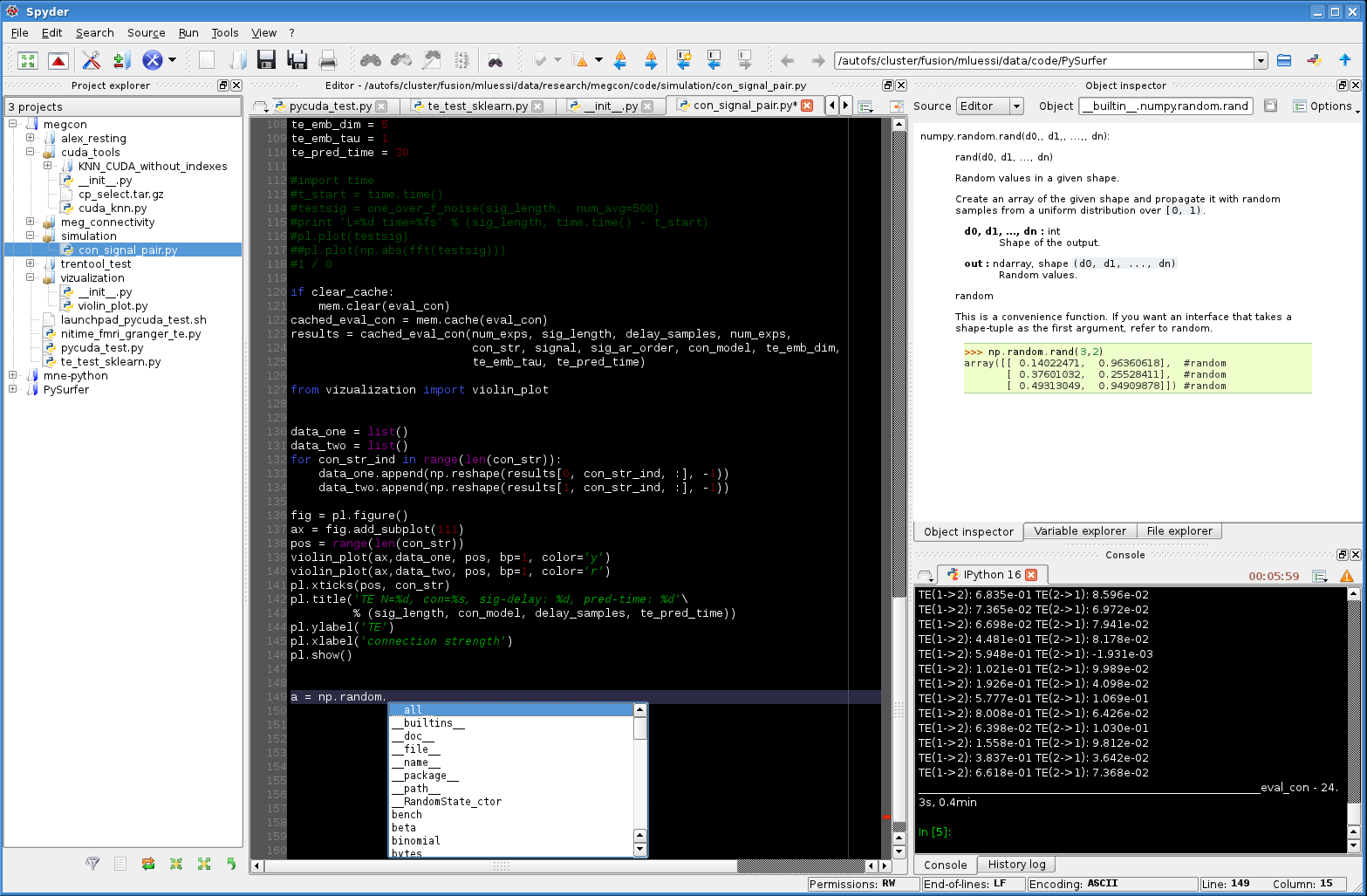PS: Spyder is written in Python and uses PySide/PyQt for the GUI

# Python basics: Numerical types

Integer variables:

```>>> 1 + 1
2
>>> a = 4
```

floats:

```>>> c = 2.1
```

complex (a native type in Python!):

```>>> a = 1.5 + 0.5j
>>> a.real
1.5
>>> a.imag
0.5
```

# Python basics: Numerical types

and booleans:

```>>> 3 < 4
True
>>> test = (3 > 4)
>>> test
False
>>> type(test)
<type 'bool'>
```

Note that you don't need to specify the type of the variable

```int a = 1;  # in C
```

# Python basics: Numerical types

Python can replace your pocket calculator with : +, -, *, /, % (modulo)

```>>> 7 * 3.
21.0
>>> 2**10
1024
>>> 8 % 3
2
```

WARNING : Integer division

```>>> 3 / 2  # !!!
1
>>> 3 / 2.  # Trick: use floats
1.5
>>> 3 / float(2)  # type conversion
1.5
```

# Strings

```>>> a = "hello, world!"
>>> print a
'l'
```
• String substitution:
```>>> 'An integer: %i; a float: %f; a string: %s' % (1, 0.1, 'string')
'An integer: 1; a float: 0.100000; a string: string'
```

Behaves very much like printf in C

```>>> print "%03d" % 2  # print fixed size
"002"
```

# Container types: list

The list type:

```>>> a = 
```

Or

```>>> a = list()
>>> a.append(1)

```

Concatenation and access:

```>>> a + a  # concatenation
[1, 1]
>>> a = 2  # access 1st element (starts at 0!)
[2, 1]
>>> a[-1] = 0  # access last element
[2, 0]
```

# Container types: list

• Slicing: obtaining sublists of regularly-spaced elements
```>>> l = [1, 2, 3, 4, 5]
>>> l[2:4]
[3, 4]
```

Note that i is in l[start:stop] if start <= i < stop

So that len(l[start:stop]) == (stop - start)

Slicing syntax: l[start:stop:stride]

```>>> l[:3]  # first 3 : in Matlab l(1:3)
[1, 2, 3]
>>> l[3:]  # from 3 to end : in Matlab l(4:end)
[4, 5]
>>> l[::2]  # every 2 element : in Matlab l(1:2:end)
[1, 3, 5]
>>> l[::-1]  # reverse list : in Matlab l(end:-1:1)
[5, 4, 3, 2, 1]
```

# Container types: dictionary

A dictionary dict is basically an efficient table that maps keys to values. It is an unordered container:

```>>> phone = {'matti': 5752, 'riitta': 5578}
>>> phone['alex'] = 5915
>>> phone
{'riitta': 5578, 'alex': 5915, 'matti': 5752}  # no order
>>> phone['riitta']
5578
>>> phone.keys()
['riitta', 'alex', 'matti']
>>> phone.values()
[5578, 5915, 5752]
>>> 'matti' in phone
True
```

# Getting help

Using the built-in help in IPython:

```>>> l = list()
>>> l.sort?  # don't forget the ?
Type:       builtin_function_or_method
Base Class: <type 'builtin_function_or_method'>
String Form:<built-in method sort of list object at 0x660ef30>
Namespace:  Interactive
Docstring:
L.sort(cmp=None, key=None, reverse=False) -- stable sort *IN PLACE*;
cmp(x, y) -> -1, 0, 1
```

# Basics of control flow: Conditional statements

```>>> a = 10
>>> if a == 1:
>>>     print(1)
>>> elif a == 2:
>>>     print(2)
>>> else:
>>>     print('A lot')
```

Blocks are delimited by indentation

# Basics of control flow: Loops

```>>> for word in ['cool', 'powerful', 'readable']:
>>>     print('Python is %s' % word)
>>>
Python is cool
Python is powerful
```

you can iterate over lists, arrays, dict etc.

# My first function

```>>> def disk_area(radius):
>>>
>>> disk_area(1.5)
7.0649999999999995
```

# My second function

Arguments are not copied when passed to a function (not like with Matlab)

```>>> def foo(a):
>>>     a.append(1)
>>>
>>> a = 
>>> foo(a)
>>> print a  # a has been modified !!!
[0, 1]
```

# NumPy

NumPy is:

• an extension package to Python for multidimensional arrays (matrices in n-dimensions)
• designed for efficient scientific computation

Example:

```>>> import numpy as np
>>> a = np.array([0, 1, 2, 3])
>>> a
array([0, 1, 2, 3])
```

Reference documentation: http://docs.scipy.org/doc/numpy/reference or: http://scipy-lectures.github.com/intro/numpy/numpy.html

# NumPy: Creating arrays

• 1-D
```>>> a = np.array([0, 1, 2, 3])
>>> a
array([0, 1, 2, 3])
```

Getting the size and dimensions of the array:

```>>> a.ndim  # in Matlab `ndims(a)`
1
>>> a.shape  # in Matlab `size(a)`
(4,)
>>> len(a)  # in Matlab `size(a, 1)`
4
```

# NumPy: Creating arrays

• 2-D
```>>> b = np.array([[0, 1, 2], [3, 4, 5]])    # 2 x 3 array
>>> b
array([[ 0,  1,  2],
[ 3,  4,  5]])
>>> b.ndim
2
>>> b.shape  # in Matlab `size(b)`
(2, 3)
>>> len(b)  # get size of the first dimension. In Matlab `size(b, 1)`
2
```
• 3-D, ...

# NumPy: Creating arrays

• Evenly spaced:
```>>> import numpy as np
>>> a = np.arange(10) # 0 .. n-1  (!)
>>> a
array([0, 1, 2, 3, 4, 5, 6, 7, 8, 9])
>>> b = np.arange(1, 9, 2) # start, end (exlusive), step
>>> b
array([1, 3, 5, 7])
```
• or by number of points:
```>>> c = np.linspace(0, 1, 6)   # start, end, num-points
>>> c
array([ 0. ,  0.2,  0.4,  0.6,  0.8,  1. ])
```

# NumPy: Creating arrays

• Common arrays: ones, zeros and eye (like in Matlab)
```>>> a = np.ones((3, 3))
>>> a
array([[ 1.,  1.,  1.],
[ 1.,  1.,  1.],
[ 1.,  1.,  1.]])
```
```>>> b = np.zeros((2, 2))
>>> b
array([[ 0.,  0.],
[ 0.,  0.]])
```
```>>> c = np.eye(3)
>>> c
array([[ 1.,  0.,  0.],
[ 0.,  1.,  0.],
[ 0.,  0.,  1.]])
```
• Random arrays
```>>> d = np.random.randn(2, 2)
>>> d
array([[-0.95731365, -0.30260599],
[ 0.43354227, -1.09239752]])
```

# NumPy: Indexing and slicing

```>>> a = np.diag(np.arange(3))
>>> a
array([[0, 0, 0],
[0, 1, 0],
[0, 0, 2]])
>>> a[1, 1]
1
>>> a[2, 1] = 10  # third line, second column
>>> a
array([[ 0,  0,  0],
[ 0,  1,  0],
[ 0, 10,  2]])
>>> a  # takes the entire second row !
array([0, 1, 0])
```

# NumPy: Indexing and slicing

Like Python lists arrays can be sliced:

```>>> a = np.arange(10)
>>> a
array([0, 1, 2, 3, 4, 5, 6, 7, 8, 9])
>>> a[2:9:3]  # [start:end:step]
array([2, 5, 8])
>>> a[::2]  # every 2 elements
array([0, 2, 4, 6, 8])
```

# NumPy: Copies and views

• A slicing operation creates a view on the original array
```>>> a = np.arange(10)
>>> a
array([0, 1, 2, 3, 4, 5, 6, 7, 8, 9])
>>> b = a[::2]; b
array([0, 2, 4, 6, 8])
```
• The original array is not copied in memory: when modifying the view, the original array is modified as well.
```>>> b = 12
>>> b
array([12,  2,  4,  6,  8])
>>> a   # no copy !!!
array([12,  1,  2,  3,  4,  5,  6,  7,  8,  9])
```

# NumPy: Copies and views

If you want a copy you have to specify it:

```>>> a = np.arange(10)
>>> b = a[::2].copy()  # force a copy
>>> b = 12
>>> a
array([0, 1, 2, 3, 4, 5, 6, 7, 8, 9])
```

This behavior can be surprising at first sight...

but it allows to save both memory and time.

# NumPy: Linear algebra

Matrix multiplication:

```>>> a = np.triu(np.ones((3, 3)), 1)   # see help(np.triu)
>>> a
array([[ 0.,  1.,  1.],
[ 0.,  0.,  1.],
[ 0.,  0.,  0.]])
>>> b = np.diag([1, 2, 3])
>>> a.dot(b)  # same as np.dot(a, b)
array([[ 0.,  2.,  3.],
[ 0.,  0.,  3.],
[ 0.,  0.,  0.]])
```

WARNING: Element-wise multiplication vs. matrix multiplication

```>>> a * b  # element-wise multiplication
array([[0, 0, 0],
[0, 0, 0],
[0, 0, 0]])
```

Transpose:

```>>> a_transposed = a.T  # no copy !
```

# NumPy: Linear algebra

Inverse, systems of linear equations and SVD:

```>>> from numpy import linalg  # OR
>>> from scipy import linalg  # even better
>>> A = a + b
>>> A
array([[ 1.,  1.,  1.],
[ 0.,  2.,  1.],
[ 0.,  0.,  3.]])
>>> B = linalg.inv(A)
>>> B.dot(A)
array([[ 1.,  0.,  0.],
[ 0.,  1.,  0.],
[ 0.,  0.,  1.]])
>>> x = linalg.solve(A, [1, 2, 3])  # linear system
>>> U, s, V = linalg.svd(A)  # SVD
>>> vals = linalg.eigvals(A)  # Eigenvalues
```

# NumPy: Reductions

Computing sums:

```>>> x = np.array([1, 2, 3, 4])
>>> np.sum(x)  # or x.sum()
10
```

Sum by rows and by columns:

```>>> x = np.array([[1, 1], [2, 2]])
>>> x.sum(axis=0)   # columns (first dimension)
array([3, 3])
>>> x[:,0].sum(), x[:,1].sum()
(3, 3)
>>> x.sum(axis=1)   # rows (second dimension)
array([2, 4])
```

Same with np.mean, np.argmax, np.argmin, np.min, np.max, np.cumsum, np.sort etc.

# Visualization with Python

```>>> import pylab as pl
>>> t = np.linspace(0, 8 * np.pi, 1000)
>>> x = np.sin(t)
>>> pl.plot(t, x)
>>> pl.xlabel('Time')
>>> pl.ylabel('Amplitude')
>>> pl.ylim([-1.5, 1.5])
>>> pl.show()
>>> pl.savefig('pylab_demo.pdf')  # natively save pdf, svg, png etc.
```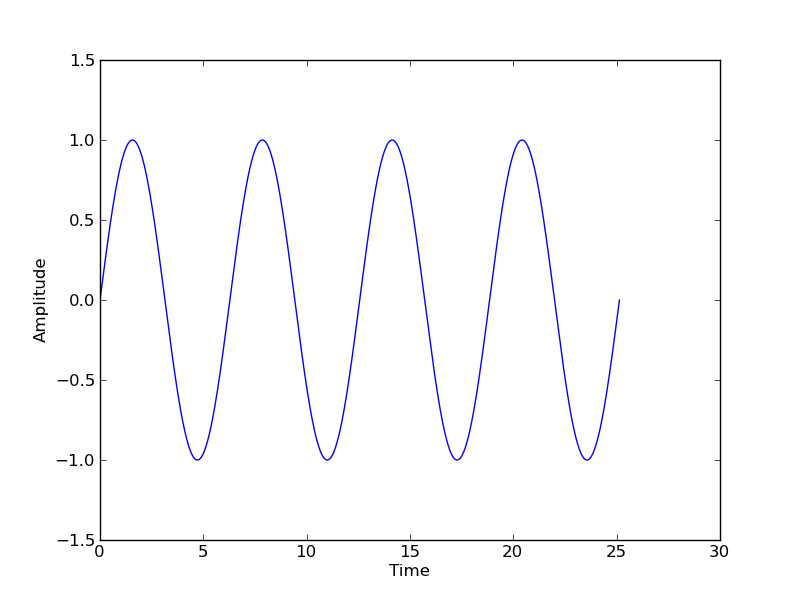# Visualization with Python

• 2-D (such as images)
```>>> image = np.random.rand(30, 30)
>>> pl.imshow(image)
>>> pl.gray()
>>> pl.show()
```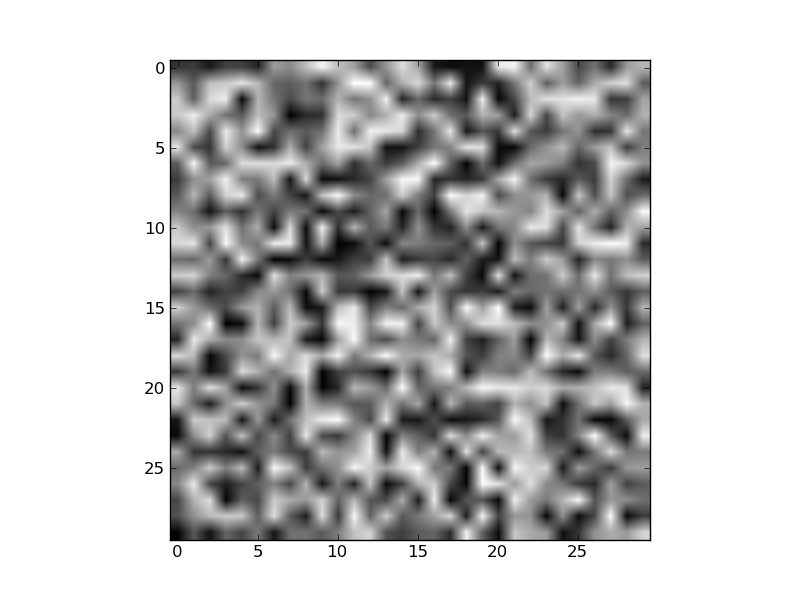# Visualization with Python

• 3-D with Mayavi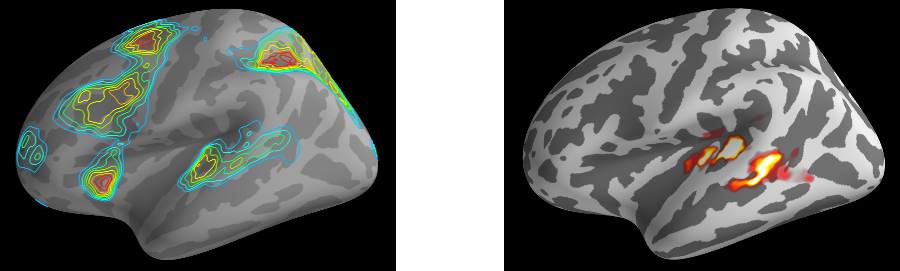Check out: http://pysurfer.github.com/

# SciPy

• scipy contains various toolboxes dedicated to common issues in scientific computing.
• scipy can be compared to other standard scientific-computing libraries, such as the GSL (GNU Scientific Library for C and C++), or Matlab's toolboxes.
• scipy is the core package for scientific routines in Python.
• scipy is meant to operate efficiently on numpy arrays.

# SciPy

• scipy.io for IO (e.g. read / write Matlab files)
• scipy.linalg for optimized linear algebra
• scipy.stats for basic stats (t-tests, simple anova, ranksum etc.)
• scipy.signal for signal processing
• scipy.sparse for sparse matrices
• scipy.fftpack for FFTs
• scipy.ndimage for N-D image processing (e.g., smoothing)
• etc.

# SciPy: example of scipy.io

```>>> from scipy import io
>>> io.savemat('file.mat', struct)
```

# SciPy: example of scipy.stats

A T-test to decide whether the two sets of observations have different means:

```>>> a = np.random.normal(0, 1, size=100)
>>> b = np.random.normal(1, 1, size=10)
>>> stats.ttest_ind(a, b)
(-2.389876434401887, 0.018586471712806949)
```

The resulting output is composed of:

• The T statistic value
• the p value